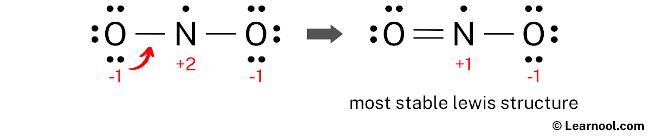# NO2 Lewis structure

The NO2 Lewis structure illustrates the arrangement of atoms in nitrogen dioxide, which consists of one nitrogen atom and two oxygen atoms. The nitrogen atom is positioned at the center and is surrounded by two oxygen atoms. One of the oxygen atoms is bonded to the nitrogen atom with a double bond, and the other oxygen atom is bonded with a single bond. The oxygen atom with the double bond has two lone pairs, while the oxygen atom with the single bond has three lone pairs. Additionally, the nitrogen atom has one unpaired electron. The nitrogen atom in the NO2 Lewis structure carries a positive charge of +1, and the oxygen atom bonded by a single bond carries a negative charge of -1.

To begin drawing the NO2 Lewis structure, it is recommended to follow a systematic approach. First, sketch a rough skeleton structure of the molecule. Then, identify the positions of the lone pairs on the atoms and assign formal charges, if required. Subsequently, minimize the formal charges by converting lone pairs on the atoms and aim for a stable Lewis structure. Repeat the process of minimizing formal charges, if necessary, until all formal charges are minimized, to ensure that the most optimal structure is achieved.

Contents

## Steps

### Sketch the structure

To sketch the NO2 Lewis structure, the first step is to determine the total number of valence electrons in the molecule. This can be calculated by multiplying the valence electrons of each atom. Nitrogen, belonging to group 15 of the periodic table, has five valence electrons, while oxygen, located in group 16, has six valence electrons. With one nitrogen atom and two oxygen atoms in NO2, the nitrogen atom contributes 5 valence electrons and each oxygen atom contributes 6 valence electrons, resulting in a total of 5 + 2(6) = 17 valence electrons.

The second step in drawing the NO2 Lewis structure is to determine the total electron pairs in the molecule. To calculate the total electron pairs, divide the number of valence electrons by two. In the case of NO2, which has a total of 17 valence electrons, the calculation would be 17 ÷ 2 = 8.5. Since a half electron pair is not possible, NO2 has a total of 8 electron pairs and one unpaired electron.

The third step involves determining the central atom, which should be the least electronegative atom. Nitrogen is less electronegative than oxygen, so it is assumed to be the central atom in NO2. Nitrogen should be placed in the center and oxygen atoms should be placed on either side. Once the central atom is determined, a rough sketch of the NO2 Lewis structure can be drawn.

### Indicate lone pair

After drawing the rough sketch of the NO2 Lewis structure, the next step is to mark the lone pairs. With a total of 8 electron pairs and one unpaired electron, and with two N – O bonds already shown, which account for the utilization of two electron pairs, it is recommended to mark the remaining six electron pairs and one unpaired electron as lone pairs on the sketch. This will help to accurately represent the electron distribution and achieve the most stable Lewis structure for NO2.

It is important to keep in mind that nitrogen and oxygen are both period 2 elements and thus, have a maximum capacity of 8 electrons in their outermost shell.

When marking the lone pairs in NO2, it is important to begin with the outer atoms, which in this case are the two oxygen atoms. Each oxygen atom will receive three lone pairs, while the nitrogen atom will receive one unpaired electron. With this in mind, the lone pairs should be marked on the sketch according to their respective atoms.

### Assign formal charge

To assign formal charges to each atom in the NO2 molecule, the following formula should be used: Formal charge = valence electrons – nonbonding electrons – ½ bonding electrons. For the nitrogen atom, the formal charge is calculated as 5 (valence electrons) – 1 (nonbonding electron) – ½ (4 bonding electrons), which gives a value of +2. Similarly, for each oxygen atom, the formal charge is calculated as 6 (valence electrons) – 6 (nonbonding electrons) – ½ (2 bonding electrons), resulting in a value of -1 for each oxygen atom.

 Atom Valence electron Nonbonding electron Bonding electron Formal charge Nitrogen 5 1 4 +2 Oxygen 6 6 2 -1

To complete the process of assigning formal charges to the NO2 molecule, it is important to mark the charges on the corresponding nitrogen and oxygen atoms in the sketch.

Due to the presence of formal charges on both nitrogen and oxygen atoms, the current Lewis structure of NO2 is unstable. In order to achieve a more stable structure, it is necessary to convert some of the lone pairs on these atoms to bonds. This will help reduce the formal charges and result in a more stable arrangement of electrons.

### Minimize formal charge

To minimize formal charges, convert a lone pair of one of the oxygen atoms to form a new N – O bond with the nitrogen atom.Lone pair of left oxygen is converted, and got the most stable Lewis structure of NO2

The final NO2 Lewis structure is unstable because the central nitrogen atom does not have a complete octet, and there are formal charges on the atoms. Although converting a lone pair to a bond could reduce the formal charges, it is not possible as nitrogen cannot accommodate more than eight electrons in its valence shell. As a result, the structure does not satisfy the octet rule. However, the formal charges on the atoms are closer to zero in this structure, making it more stable than previous ones. Therefore, this Lewis structure can be considered as the most stable structure for NO2.

Next: NH3 Lewis structure